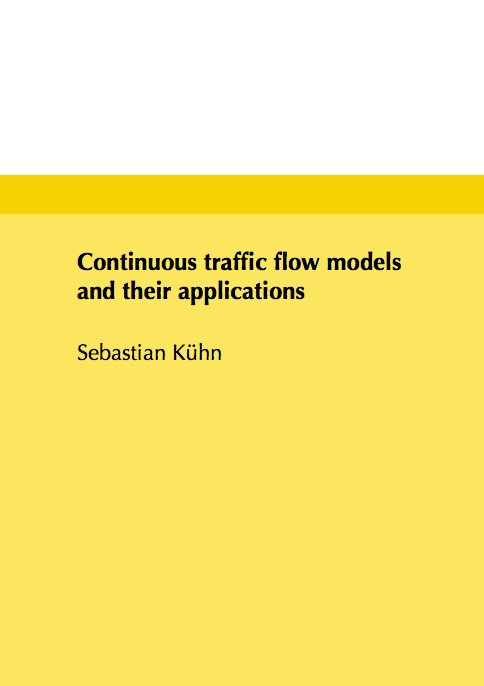Datenbestand vom 25. Juli 2020aktualisiert am 25. Juli 2020

# ISBN 978384392231996,00 € inkl. MwSt, zzgl. Versand

978-3-8439-2231-9, Reihe Mathematik

Sebastian Kühn
Continuous traffic flow models and their applications

135 Seiten, Dissertation Technische Universität Kaiserslautern (2014), Softcover, B5

## Zusammenfassung / Abstract

In this work we investigate macroscopic traffic flow models and the derivation of evacuation routes therein.

After reviewing the traffic flow model in use, we devise a general optimization problem yielding a system optimal distribution of traffic within a network. We present two different first-discretize-then-optimize approaches to numerically solve the optimization problem defined before: an adjoint approach and a mixed integer programming approach. We formally proof the connection between the two numerical approaches using dual programming.

Furthermore, we present some numerical examples, which give rise to an enhanced numerical solution technique for mixed integer programs using dual programming.

Then, we extend the traffic flow model by queues which model the dynamics at the intersections of the roads. We show that with this extension the model is not limited to traffic flow, but can also be applied to describe the flow in production networks.

We further extend the model by deriving a framework to include stochasticity, i.e. random blockages and clearances of roads, in the traffic flow network. The framework which we present is very general and can also be applied e.g. to production networks. Thus, we consider a flow line as a numerical example. By comparing our model to a discrete event simulation we are able to show the high accuracy of our model.

Furthermore, we are able to quantify the transient effects of increasing and decreasing inventory levels over time as well as to gain new insights regarding time-dependent effects in flow lines.

In the last part of this work, we consider evacuation dynamics under the influence of a gaseous hazard. Therefore we introduce dispersion models for the hazard, which we couple to the traffic flow model. This is a new way to handle evacuation dynamics and has not considered before. We present an optimization problem for the computation of optimal evacuation routes.

In the numerical examples we show, that the presented traffic flow model is more appropriate to model real world scenarios than standard evacuation models as it is capable of handling congestion properly. We also show that the standard objective function for the computation of evacuation routes, i.e. the quickest flow, is not sufficient when considering the evacuation due to a gaseous hazard. For this case we present two alternative objective functions and show that they lead to a safer evacuation.